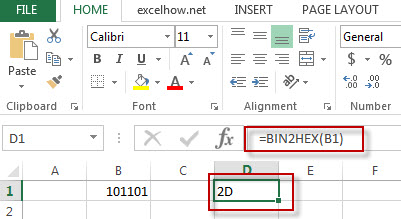# Excel Bin2Hex Function

This post will guide you how to use Excel BIN2HEX function with syntax and examples in Microsoft excel.

### Description

The Excel BIN2HEX function converts a binary number to hexadecimal.

The BIN2HEX function is a build-in function in Microsoft Excel and it is categorized as an Engineering Function.

The BIN2HEX function is available in Excel 2016, Excel 2013, Excel 2010, Excel 2007, Excel 2003, Excel XP, Excel 2000, Excel 2011 for Mac.

### Syntax

The syntax of the BIN2HEX function is as below:

= BIN2HEX (binary_number)

Where the BIN2HEX function argument is:
Binary_number -This is a required argument. The binary number that you want to convert to hexadecimal.

### Example

The below examples will show you how to use Excel BIN2HEX Function to convert a binary number to a hexadecimal number.

#1 = BIN2HEX (B1)Related Posts

Excel Complex Function

This post will guide you how to use Excel COMPLEX function with syntax and examples in Microsoft excel. Description The Excel COMPLEX function converts real and imaginary coefficients into a complex number. The COMPLEX function is a build-in function in ...

Excel Bin2Oct Function

This post will guide you how to use Excel BIN2OCT function with syntax and examples in Microsoft excel. Description The Excel BIN2OCT function converts a binary number to octal. The BIN2OCT function is a build-in function in Microsoft Excel and ...

Excel Bin2Dec Function

This post will guide you how to use Excel BIN2DEC function with syntax and examples in Microsoft excel. Description The Excel BIN2DEC function converts a binary number to decimal. The BIN2DEC function is a build-in function in Microsoft Excel and it ...

Sidebar